# Kerala Syllabus 9th Standard Physics Solutions Chapter 3 Motion and Laws of Motion

You can Download Motion and Laws of Motion Questions and Answers, Summary, Activity, Notes, Kerala Syllabus 9th Standard Physics Solutions Part 1 Chapter 3 help you to revise complete Syllabus and score more marks in your examinations.

## Kerala State Syllabus 9th Standard Physics Solutions Chapter 3 Motion and Laws of Motion

### Motion and Laws of Motion Textual Questions and Answers

Question 1.
What will be the result when a man tries to move a vehicle by pushing it, standing inside the vehicle? The vehicle moves/the vehicle doesn’t move.
The vehicle doesn’t move.Question 2.
What if the same vehicle is pushed from outside.
Yes, the vehicle moves
Internal forces can not move an object, but only an external unbalanced force can cause motion.

Newton’S First Law Of Motion

Every object continues in its state of rest or of uniform motion along a straight line unless an unbalanced external force acts on it. This is Newton’s First law of motion.

Question 3.
What is inertia of rest?
Inertia of rest is the tendency of a body to remain in its state of rest or its inability to change its state of rest by itself.

Question 4.
Write down an activity to show inertia of rest.Place a bottle filled with water on a thick rough pa¬per as shown. Pull the paper suddenly to one side. The bottle falls in the opposite direction fo the motion of paper. This is because of inertia of rest.

Question 5.
What is inertia of motion?
Inertia of motion is defined as the inability of a body to change it state of motion by itself.

Question 6.
Write down an activity to show inertia of motion.Place a bottle on a thick paper with rough surface. Bring the bottle into motion by pulling the paper slowly. Gradually increase the speed of pulling stop pulling when the bottle gains a certain speed. The bottle falls in the direction of motion of the pa-per, due to inertia of motion of the bottle.

Question 7.
Find out reasons for the Situations
a) Place some carom board coins in a pile. Using the striker, strike out the coin at the bottom. What do you observe? What is the reason?
b) When a running bus is suddenly stopped, passengers, standing in the bus show a tendency to fall forward.
c) Place a small brick on a plank. When the plank
is pulled suddenly the brick remains in the same position as before.
d) When a bus moves forward suddenly from rest, the standing passengers tend to fall backward.
e) Accidents that happen to passengers who do not wear seat belts are more fatal.
a) Only the coin at the bottom is thrown away. Others will remain in the previous stage.
b) The passengers start to move forward due to the tendency to continue in its state of motion.
c) It is due to the tendency to continue in the state of rest.
d) The passengers tend to fall backward due to the tendency to continue in their state of rest.
e) The passengers inside the vehicle have the tendency to move forward due to inertia of motion.
Inertia: The inability of a body to change its state of rest or of uniform motion along a straight line by its itself.
Inertia of rest: Inertia of rest is defined as the inability of a body to change its state of rest by itself.
Inertia of motion : Inertia of motion is defined as the inability of a body to change its state of motion by itself. Eg. When a running bus is suddenly stopped, the standing passengers fall forward due to inertia of motion. When a bus at rest starts suddenly, the standing passengers fall backward due to inertia of rest.

Question 8.
Expand the table by finding more situations from daily life.Inertia of rest Inertia of motion 1. When the branch of a mango tree is shaken mangoes fall. 1. A running athlete cannot stop himself abruptly at the finishing point. 2. When the carpet is trapped, dust particle scatters. 2. A man stepping down from a slowly moving bus stops after few steps of running. 3. When the coin on a Cardboard placed over a glass is struck, it falls into the glass 3. In hammer throw, before the hammer is let go off, it is whirled along a circular path.

Question 9.
Find out the reasons
a) An athlete doing a long jump-start his run from a distance.
b) A running elephant cannot change its direction suddenly.
a) This activity helps to cover long distances by utilizing inertia of motion.
b) Mass of elephant is greater. So inertia of motion also be greater. Also, it cannot be able to change its direction suddenly.

Mass And Inertia

Question 10.
It is dangerous for loaded vehicles to negotiate a curve in the road without reducing speed. What is the reason?
Loaded vehicles possess more inertia of motion. As ” mass increases, inertia also increases.Question 11.
It is more difficult to roll a filled tar drum than an empty drum.
a) Which of two has a greater mass?
b) Which has greater inertia?
a) The mass of drum filled with tar will be greater,
b) Inertia will be greater to tar filled drum
Conclusion: Inertia depends on mass. When the mass increases inertia also increases. When the mass decreases inertia also decreases.

Question 12.
If a tennis ball (mass 58.5 g) and a cricket ball (mass 163 g) are the reach a certain distance when hit with a cricket bat, which is to be hit with greater force? The tennis ball / the cricket ball?
The cricket ball

Question 13.
Will the change of velocity be the same in both the cases?
Velocities are different in both cases due to the difference in masses.

The inertia of an object depends upon its mass. When the mass increases, inertia also increases.

Momentum

Momentum is a characteristic property of moving objects. It is measured as the product of the mass of the body and its velocity.

Momentum = mass × velocity
Unit of momentum is kg m/s

Question 14.
A car of 1000 kg moves with a velocity of 10 m/s. On applying brakes it comes to rest in 5s. Then what are its initial momentum and final momentum?
m = 1000 kg
u = 10 m/s
v = 0
t = 5s
Initial momentum = mu
= 1000 × 10 =10000 kg m/s
Final momentum = mV
= 1000 × 0 = 0 Kg m/s

Question 15.
A hockey ball of mass 200 g hits on a hockey stick with a velocity 10 m/s. Calculate the change in momentum if the ball bounces back on the same path with the same speed.
m = 200g = 200/1000 = 0.2 kg
Initial momentum = mu
= 0.2 × 10 = 2 kg m/s
Final momentum = mv
= 0.2 × 70 = 2 kgm/s
change in momentum = mv – mv = – 2 – 2
= -4 kg m/s

Question 16.
A loaded lorry of mass 12000 kg moves with a velocity of 12 m/s. Its velocity becomes 10 m/s after 5 s.
a) What is the initial momentum and what is the final momentum?
b) What is the change in momentum?
c) What is the rate of change of momentum?
a) Initial momentum = mu
=12000 × 12 = 144000 kg m/s Final momentum = mv
=12000 × 10 = 120000 kg m/s
b) Change in momentum = mv – mu
= 120000 – 144000 = –24000 kg m/s
c) Rate of change in momentum = $$\frac { mv – mu }{ t }$$
= $$\frac { -24000 }{ 5 }$$
= – 4800 kgm/s2

Newton’S Second Law Of Motion

The rate of change of momentum of a body is directly proportional to the unbalanced external force acting on it.Equation for Calculating Force:
According to second law of motion F ∝ ma
F = kma (k – a constant)
k = 1 ∴ F = 1 × ma
F = ma,ie. F- Force, m – mass, a – acceleration.
Unit of force is Newton (N)
Another unit is dyne.

Question 17.
A constant force is applied for 2 s on a body of mass 5 kg. As a result, if the velocity of the body is changed from 3 m/s to 7 m/s, find out the value of the applied force.Question 18.
A car moving with a speed of 108 km/h comes to rest after 4s on applying brake, if the mass of the car including the passengers is 1000 kg, what will be the force applied when brake is applied?
Initial velocity of the car u = 108 km/h= 30 m/s
Final velocity v = 0
Mass m = 1000 kg
Time t = 4s
According to newton’s second law
F = ma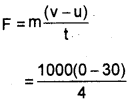= – 7500 N
The negative sign indicates that the applied force is opposite to the direction of motion.

Question 19.
Velocity of an object of mass 5 kg increases from 3 m/s to 7 m/s on applying a force continuously for 2s. Find out the force applied. If the duration for which force acts is extended to 5s, what will be the velocity of the object then?
u = 3 m/s
v = 7 m/s
t = 2s
m = 5 kg
According to Second Law of Motion F = ma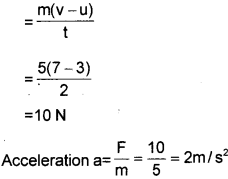If we substitute the values in the equation v = u + at, velocity can be calcualted when the time for force is extended to 5s.
v = 3 + (2 × 5) = 13 m/s

Question 20.
Velocity-time graph of an object of mass 20 g, moving along the surface of a long table is given below.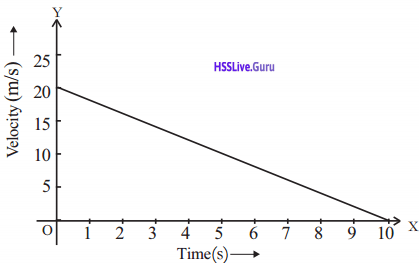What is the frictional force experienced by the object?
From the graph
Initial velocity u = 20 m/s
Final velocity v = 0m/s
t = 10 s
m = 20
g = 20/100 kg
F = ma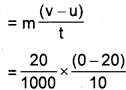= -0.04 N
The negative sign shows that the frictional force is acting opposite to the direction of motion of the object.

Question 21.
m1 and m2 are the masses of two bodies. When a force of 5 N is applied on each body, m1 gets an acceleration of 10 m/s2 and m2, 20 m/s2. If the two bodies are tied together and the same force is applied, find the acceleration of the combined system.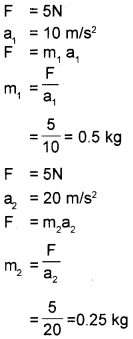Impulse

Impulsive force is a very large force acting for a very short time.
Impulse of force is the product of the force and the time.
Impulse = Force × time
Impulse – Momentum Principle
According to Newton’s second law of motion,
$$F=\frac{m(v-u)}{t}$$
F x t = m(v – u)
F × t= mv – mu
ie. impulse = change Is momentum This is known as impulse-momentum principle. It states that a change in momentum of an object is equal to the impulse experienced by it.

Question 22.
Explain the following situation by relating force and time.
When the change in momentum is a constant, the force acting on a body will be inversely proportional to the time taken. As time increase, the force acting decreases and as time decreases, the force acting increases.Question 23.
During a pole vault jump, the impact is reduced by falling on a foam bed.
When the change in momentum is a constant, the force acting on a body will be inversely proportional to the time taken. As time increase, the force acting decreases and as time decreases, the force acting increases.

Question 24.
Hay or sponges are used while packing glassware. This helps to avoid breaking of glasswares due to collision.
When the change in momentum is a constant, the force acting on a body will be inversely proportional to the time taken. As time increase, the force acting decreases and as time decreases, the force acting increases.

Question 25.
Karate experts move their hands with great speed to chop strong bricks.
When the change in momentum is a constant, the force acting on a body will be inversely proportional to the time taken. As time increase, the force acting decreases and as time decreases, the force acting increases.

Newton’S Third Law Of Motion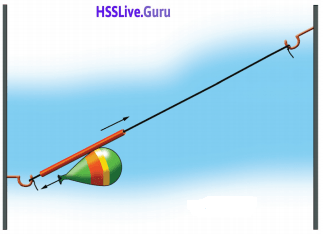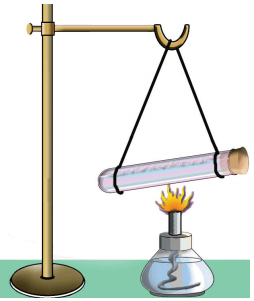Pass a long string through a straw and tie the string between two windows of the classroom Paste an inflated balloon on the straw.

Question 26.
Inflate a balloon and release it suddenly. What happens?
Release of air causes the balloon to move in the opposite direction. Releasing air from the balloon is action and the movement of the balloon is reaction.

Question 27.
What do you observe?
The cork pushed out due to the pressure of steam. The tube moves backward.

Question 28.
If the action is the cork being pushed out due to the force exerted by the steam on it, what is the reaction?
The reaction is the backward movement of boiling tube.

Question 29.
Write down the action and reaction while we are walking on a floor?
When we are walking through a floor, we applies a force on the floor. This is action. The floor applies a force in the opposite direction. This is reaction.
Rocket Propulsion:

• Escaping of hot gases from the jet of rocket is action.
• The force exerted by these gases on the rocket is reaction.

Question 30.
Are the action and reaction equal and opposite?
Action and reaction are equal and opposite.
Newton’s Third law of Motion For every action there is an equal and opposite reaction.

Question 31.
Examine the following situations and complete the table.Situation Action (FJ Reaction (FJ 1. A man jumps from a boat to ’ the shore. The man exerts a force on the boat. The boat moves backward. The boat exerts an equal force on the man. The man lands on the shore. 2. A bullet is fired from a gun the bullet exerts a force on the gun So it moves backward The gun exerts an equal force to the bullet, so it moves forward 3. A boat is rowed. The man applied force on the water The boat moves forward.

Conclusions:

• As a result of the applied force by a second body to a body, reaction will occur at the second body.
• Action and reaction are equal and opposite.
• Since action and reaction takes place in two different bodies, they do not cancel each other.

Law Of Conservation Of Momentum

Observe the figure and answer the following questions.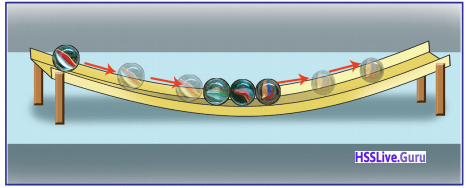Question 32.
Move the first marble slightly back and roll forward. What happens?
One marble will be thrown off from the other end and reaches the previous position.Question 33.
Bring the two marbles into contact and let them roll. What happens?
Two marbles will move from the other end.Observe the figure and answer the following questions.

Question 34.
Total momentum before collision = ………….
m1 u1 + m2 u2.

Question 35.
Total momentum after collision =…….
m1 v1 + m2 v2

Question 36.
Initial momentum of A=
m1 u1

Question 37.
Final momentum of A =
m1 v1

Question 38.
Change in momentum of A=
m1 v1 – m1 u1

Question 39.
Rate of change of momentum of A =
$$\frac{m_{1} v_{1}-m_{1} u_{1}}{t}$$

Question 40.
Initial momentum of B =……
m2 u2Question 41.
Final momentum of = …………….
m2 v2

Question 42.
Change in momentum of B = ………
m2 v2 – m2 u2.

Question 43.
Rate of change of momentum of B = ……………
$$\frac{\mathrm{m}_{2} \mathrm{v}_{2}-\mathrm{m}_{2} \mathrm{u}_{2}}{\mathrm{t}}$$

According to IInd Law of motion, rate of change of momentum is directly proportional to the external force.
Force exerted by B on A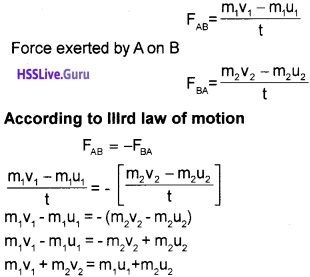Law Of Conservation Of Momentum

In the absence of an external force, the total momentum of a system is a constant.

Question 44.
A bullet is fired with a velocity v from a gun of mass M. What will be the recoil velocity of the gun if the mass of the bullet is m?
According to low of Conservation of Momentum, total momentum ofthe gun and the bullet before firing and their total momentum after firing will be equal,
ie. Total momentum before firing = 0 + 0 =0
Total momentum after firing = MV + mv
Accoriding to Law of Conservation of Momentum 0 =MV + mv
MV = -mv
V = $$\frac { -mv }{ M }$$
The recoil velocity ofthe gun V = $$\frac { -mv }{ M }$$. The negative
sign indicates that the gun moves in the opposite direction of motion ofthe bullet.

Question 45.
Suppose a child of mass 40 kg running on a horizontal surface with a velocity of 5m/s jumps on a stationary skating board of mass3 kg while running as shown in the figure. If there is no other force acting horizontally (assuming the frictional force on the wheels to be zero), calculate the velocity of the combined system of child and the skating board.
Suppose the velocity of the board while moving is u. Total momentum of the child and the skating board before jumping will be
= 40 kg × 5 m/s + 3 kg × 0 m/s
= 200 kg m/s Total momentum when the system starts moving (body and skating board)
= (40 + 3) kg × u m/s
= 43 × u kgm/s.
According to the Law of Conservation of Momentum, Total momentum remains constant.
43 u = 200
u = 200/43 = + 4.65 m/s

Circular Motion

Motion of a body through a circular path is known as circular motion.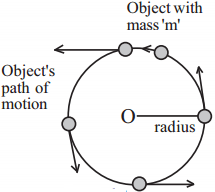Question 46.
Does the velocity of an object moving with a uniform speed along a circular path change?
Yes

Question 47.
How does this change in velocity happen?
Due to change in speed/change in direction/due to change in both speed and direction.
Due to change in direction.

• Whirling of a stone tied to a string is a type of circular motion.
• The force we apply form the center of the circle reaches the object through the string. The acceleration which a body in circular motion experiences towards the center of the circle through the radius is centripetal acceleration. The force that creates centripetal acceleration is a centripetal force.
Centripetal force (Fc) = mv2/t
m – mass, v-velocity, r- radius of the circular path
• In the absence of centripetal force, circular moving body thrown off through the tangent.
• If a body moving along a circular path covers equal distances in equal intervals of time, it is said to be in uniform circular motion.

Question 48.
In hammer throw, before the hammer is let go off, why is it whirled around along a circular path?
It is to get initial momentum. Also helpful to cover long distances through the tangent.

Question 49.
How does the speed of a giant wheel in an amusement park?
The motion of giant wheel is controlled by mechanically. So its speed is uniform except when starting and stopping.
Examples for uniform circular motion:

• Motion of needles in a watch.
• Whirling of a stone tied to a string.
• Movement of the leaf of an electric fan except when starting and stopping.
• Circular motion of earth’s artificial /satellites.

The acceleration experienced by an object in a circular motion, along the radius, towards the center of the circle, is known as centripetal acceleration. The force that creates a centripetal acceleration is called centripetal force. Centripetal acceleration and centripetal force are directed towards the center.
If an object moving along a circular path covers equal distances in equal intervals of time, it is said to be in uniform circular motion.

Let Us Assess

Question 1.
Observe the figures given below. Answer the following questions.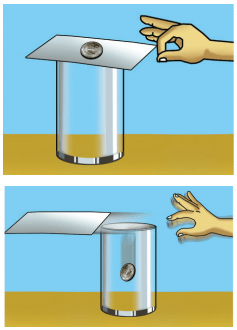a) When the card is suddenly struck off, what happens to the coin? Explain.
b) What is the law to which this property is related?
c) How is this property related to the mass of the object?
a) The coin falls into the glass due to inertia of rest.
b) Newton’s 1st law of motion.
c) Mass increases inertia increases.

Question 2.
What are the balanced forces acting on a book at rest on a table?
a) Downward force exerts by the book on the table (i.e., weight of the book).
b) Upward force applies by the table on the book (reaction).

Question 3.
To remove the dust from a carpet, it is suspended and hit with a stick. What is the scientific principle behind it?
Inertia of rest. The dust in the carpet shows the tendency to continue in its state of rest.

Question 4.
Acar and a bus are traveling with the same velocity. Which has greater momentum? Why?
Bus. Because when mass increases momentum increases.

Question 5.
On the basis of Newton’s third law of motion, explain the source of force that helps to propel a rocket upward.

• It is due to the reaction force by the escaping gas from rocket.
• Escaping gases form the rocket is action.
• The force exerted by escaping gases on the rocket is reaction.

Question 6.
A car-travels with a velocity of 15 m/s. The total mass of the car and the passengers in it is 1000 kg. Find the momentum of the car.
Momentum p = mv
= 1000 × 15
= 15000 kg m/s

Question 7.
Give reasons:
a) When a bullet is fired from a gun, the gun recoils.
b) When a bus at rest suddenly moves forward, the passengers, standing in the bus, fall backward.
c) We slip on a mossy surface.
a) The gun recoils due to the reaction force applied by the shot to the gun. Forward movement of the shot is action and the Backward movement of the gun is reaction.
b) Inertia of rest is the reason. The passengers tends continue in state of rest.
c) Absence of reaction force is the cause for this.

Extended Activities

Question 1.
Prepare and present an experiment to illustrate inertia of rest.
Make a pile of coins on a table. Strikes off the lowest coin by a knife quickly. Only that particular coin thrown off and the others remains in the previous manner. This is due to the tendency of coins to remain in its state of rest.

Question 2.
Find out situations from our daily life to explain the law of conservation of momentum and note them down.

• Recoil of a gun during firing.
• Rocket propulsion.
• Bomb explosion.

### Motion and Laws of Motion More Questions and Answers

Question 1.
Fill in the blanks.
a) As the time interval decreases, rate of change of momentum
b) The force required to produce an acceleration of 1 m/s2 on a body of mass 1 kg is
a) Increases
b) 1 Newton (1N)

Question 2.
Correct the statement if any wrong.
An object moving with uniform speed along a circular path undergoes velocity change due to the change in speed.
An object moving with a uniform speed along a circular path undergoes velocity change due to change in direction.

Question 4.
a) What is meant by momentum?
b) Write down the equation for calculating momentum.
c) A body of mass 50 kg starts from rest. If its velocity changes to 15 m/s after 10 seconds, calculate the change in momentum? What will be the rate of change of momentum?
a) The measurement of the quantity of motion of a body is called momentum
b) Momentum p = mvQuestion 5.
a) Define circular motion and uniform circular motion.
b) What is the relation between centripetal force and centripetal acceleration?
a) The motion of an object through a circular path is said to be circular motion.
If an object moving along a circular path covers equal distance in equal intervals of time if is said to be uniform circular motion.
b) The force requires to produce centripetal acceleration is centripetal force.Question 6.
a) State Newton’s II law of motion?
b) Derive the equation for force on the basis of this law.
a) The rate of change of momentum of a body is directly proportional to the unbalanced external force acting on it.
b) According to II law.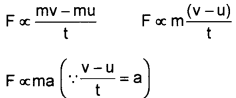F = kma (k – a constant)
The force required to produce an acceleration of 1 m/s2 on a body of mass 1 kg is 1N
1 = k × 1 × 1
k = 1
∴ F = 1 × ma
F = ma

Question 7.
A car of mass 1000 kg runs with a velocity of 10 m/s. What is the momentum of this car?
Momentum p = mv
m = 1000 kg
v = 10 m/s
∴ P = 1000 × 10
= 10000 kg m/s

Question 8.
A loaded lorry of mass 1500 kg moves with a velocity of 12 m/s. Within a small interval of the time, the velocity becomes 10 m/s.
a) What is the initial momentum of the lorry?
b) What is its final momentum?
c) What is the change in momentum?
= $$\frac { change in momentum }{ Time }$$
= $$\frac { m(v – u) }{ t }$$